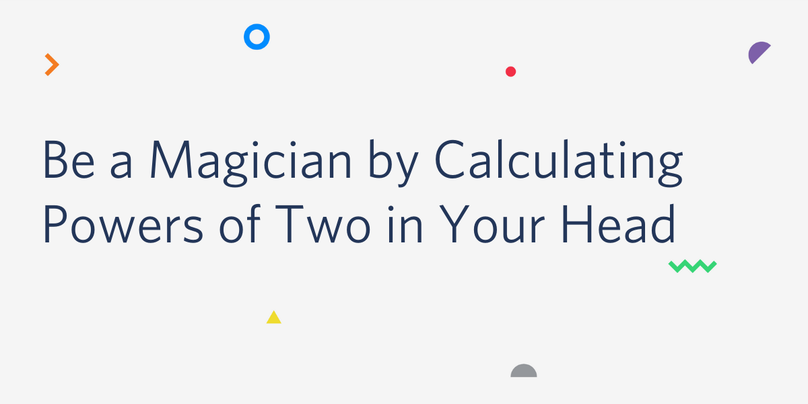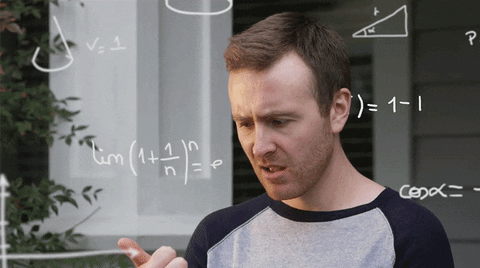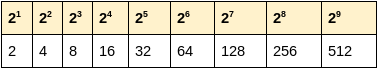﻿ Be a Magician by Calculating Powers of Two in Your Head - TwilioLevel up your Twilio API skills in TwilioQuest, an educational game for Mac, Windows, and Linux. Download Now

# Be a Magician by Calculating Powers of Two in Your HeadAs a programmer you might have to deal with questions like these:

What's bigger, 243 or 7 trillion?

or

How many items can we store if our database uses a 32-bit identifier?Calculations involving powers of two come up often in programming, and it's completely possible to work these out in your head faster than opening your calculator app, using this one (weird?) trick.

## How?

There are three things to remember:

• First, 2(a+b) = 2a × 2b
• Second, 210 is 1024, which is really close to 1000.
• Third, you need to know the powers of 2 up to 210These facts are the basis for the rule:

Add three zeros for each ten in the power, then multiply by whatever is left.

## Examples

### What’s bigger, 243 or 7 trillion?

Using the first fact we can split up the 43 into 4 tens and a three:

243 = 2(10+10+10+10+3)

= 210 × 210 × 210 × 210 × 23

Using the second, we can approximate each 210 with 1000:

243 ≅ 1000 × 1000 × 1000 × 1000 × 23

Four thousands multiply to make a trillion and the 23 is 8, so we can say that 243 is about 8 trillion, which is definitely more than 7 trillion ✔️

### How many items can we store if our database uses a 32-bit identifier?

An “n-bit” data-type can have 2n different values, so we can use the same procedure as before:

232 = 2(10+10+10+2)

= 210 × 210 × 210 × 22

So

232 ≅ 1000 × 1000 × 1000 × 22

which is 4 billion ✔️

## Recap

Add three zeros for each ten in the power, then multiply by whatever is left.

You can stop there if you are happy with your approximation being a few percent off. This is often good enough, but there’s another step you can do in your head if you need to.

## Hang on, 1024 != 1000

You are correct. It is 2.4% more. So this method is good for a quick approximation but it will always underestimate the true answer. By how much? By about 2.4% per 210. So you can get a better approximation by adding 2.5% (ie a quarter of 10%) for each 210 in your calculation.

For example, our approximation of 243 as 8 trillion is going to be about 4 x 2.5% = 10% too low. Add ten percent to make 8.8 trillion and you’re within 0.05% of the real answer (which is 8.796 trillion) ✔️

## What Next?

I first learned this trick from Samy Kamkar in a 2018 DefCon talk, and have been able to use it several times since (I've linked to the right part of the talk, but note that the full talk has some images on the slides that might not be safe for your work). Practise a few times and you'll be able to do it in less time than it would take to open your calculator app - and you can impress people at parties too!

What other quick mental shortcuts do you use? Let me know in the comments below or on twitter @MaximumGilliard.

Authors
•Matthew Gilliard
Not ready yet? Talk to an expert.